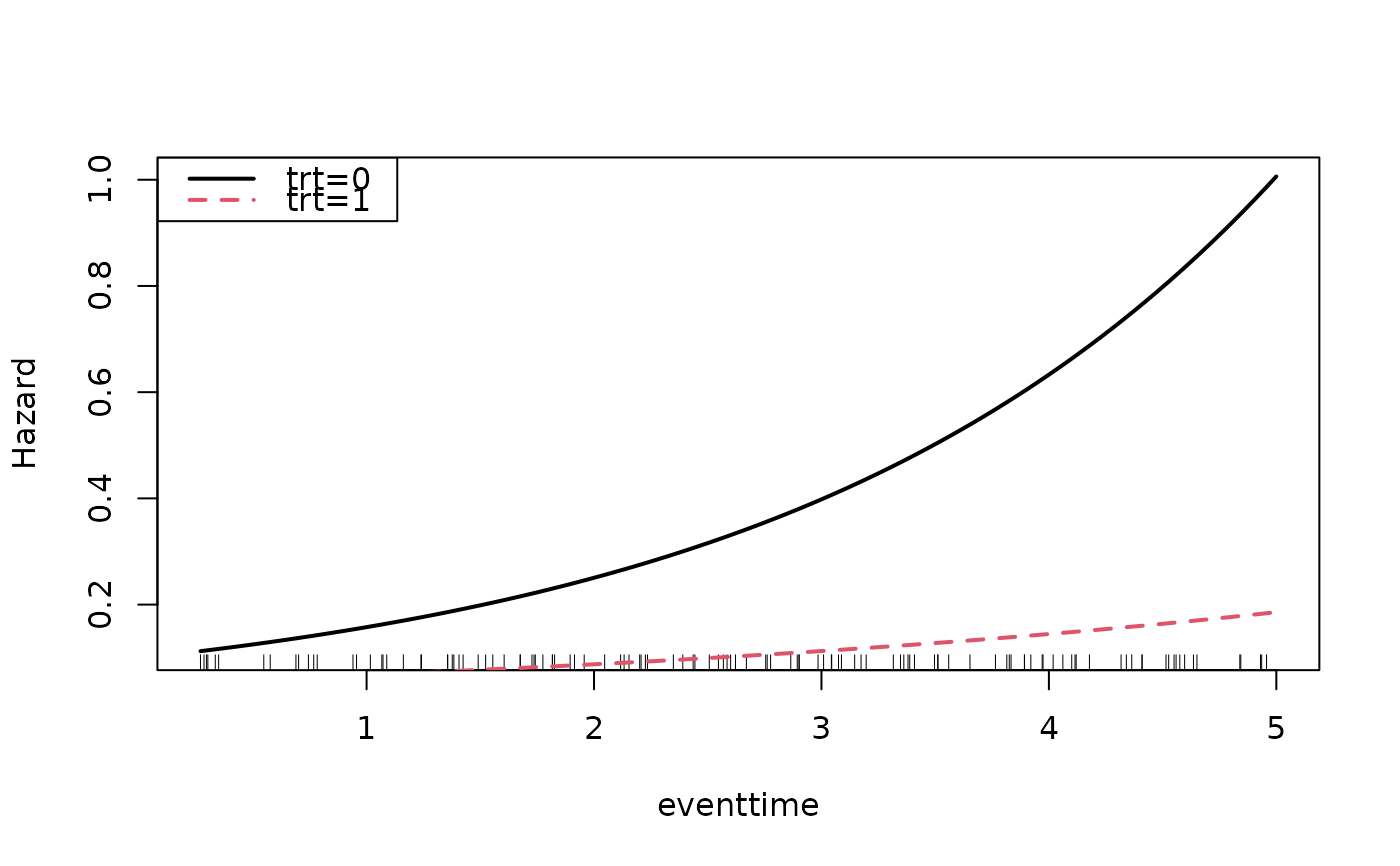Visualize estimated hazard curves as a function of time with confidence intervals. This function takes as input, the result from the casebase::fitSmoothHazard() function. The user can also specify a sequence of times at which to estimate the hazard function. These plots are useful to visualize the non-proportional hazards, i.e., time dependent interactions with a covariate.

hazardPlot(
object,
newdata,
type = c("hazard"),
xlab = NULL,
breaks = 100,
ci.lvl = 0.95,
ylab = NULL,
line.col = 1,
ci.col = "grey",
lty = par("lty"),
s = c("lambda.1se", "lambda.min"),
times = NULL,
...
)

## Arguments

object Fitted object of class glm, gam, cv.glmnet or gbm. This is the result from the casebase::fitSmoothHazard() function. A data frame in which to look for variables with which to predict. This is required and must contain all the variables used in the model. Only one covariate profile can be used. If more than one row is provided, only the first row will be used. Type of plot. Currently, only "hazard" has been implemented. Default: c("hazard") x-axis label. Default: the name of the time variable from the fitted object. Number of points at which to estimate the hazard. This argument is only used if argument times=NULL. This function will calculate a sequence of times between the minimum and maximum of observed event times. Default: 100. Confidence level. Must be in (0,1), Default: 0.95 y-axis label. Default: NULL which means the function will put sensible defaults. Line color, Default: 1. See graphics::par() for details. Confidence band color. Only used if argument ci=TRUE, Default: 'grey' Line type. See graphics::par() for details, Default: par("lty") Logical; if TRUE add to an already existing plot; Default: FALSE Logical; if TRUE confidence bands are calculated. Only available for family="glm" and family="gam", Default: !add Logical. Adds a rug representation (1-d plot) of the event times (only for status=1), Default: !add Value of the penalty parameter lambda at which predictions are required (for class cv.glmnet only). Only the first entry will be used if more than one numeric value is provided, Default: c("lambda.1se", "lambda.min") Vector of numeric values at which the hazard should be calculated. Default: NULL which means this function will use the minimum and maximum of observed event times with the breaks argument. further arguments passed to graphics::matplot()

## Value

a plot of the hazard function and a data.frame of original data used in the fitting along with the data used to create the plots including predictedhazard which is the predicted hazard for a given covariate pattern and time predictedloghazard is the predicted hazard on the log scale. lowerbound and upperbound are the lower and upper confidence interval bounds on the hazard scale (i.e. used to plot the confidence bands). standarderror is the standard error of the log hazard (only if family="glm" or family="gam")

## Details

This is an earlier version of a function to plot hazards. We recommend instead using the plot method for objects returned by casebase::fitSmoothHazard(). See casebase::plot.singleEventCB().

casebase::fitSmoothHazard()

## Examples

data("simdat")
mod_cb <- fitSmoothHazard(status ~ trt * eventtime,
time = "eventtime",
data = simdat[1:200,],
ratio = 1,
family = "glm")

results0 <- hazardPlot(object = mod_cb, newdata = data.frame(trt = 0),
ci.lvl = 0.95, ci = FALSE, lty = 1, line.col = 1, lwd = 2)head(results0)#>     trt eventtime offset predictedloghazard predictedhazard
#> 1     0 0.2695865      0          -2.186588       0.1122993
#> 1.1   0 0.3173684      0          -2.164438       0.1148144
#> 1.2   0 0.3651504      0          -2.142289       0.1173859
#> 1.3   0 0.4129323      0          -2.120139       0.1200149
#> 1.4   0 0.4607143      0          -2.097989       0.1227029
#> 1.5   0 0.5084963      0          -2.075840       0.1254510hazardPlot(object = mod_cb, newdata = data.frame(trt = 1), ci = FALSE,
ci.lvl = 0.95, add = TRUE, lty = 2, line.col = 2, lwd = 2)legend("topleft", c("trt=0","trt=1"),lty=1:2,col=1:2,bty="y", lwd = 2)